Warning: Illegal string offset 'html' in /home/hsn/public_html/forum/cache/skin_cache/cacheid_1/skin_topic.php on line 909

cirlces help please - HSN forum2 replies to this topic

### #1xlynsey

• Gender:Female

Posted 06 May 2007 - 05:59 PM

hi there
im stuck on this q:
in each question find the equation of the circle from the info given:

1)
a circle passes thru the origin and the point (30,0) and has the line y= -5 as a tangent.

2) the line y=1 is a tangent to the cirlce which passes through (-1,0)and (5,0)

and theres 4 more of this format, i just cannot think how to do them!!!

lynseyTop of the Class

•• Members
•• 390 posts
• Location:Cambridge
• Interests:Muzak.
• Gender:Male

Posted 06 May 2007 - 08:17 PM

I can't remember getting any questions like this at higher, where did you come across it? If these are exam questions, then there's probably a quicker way to do this that I haven't noticed here.

Anyway, for the first one, here's a (rather longwinded) sketch of a solution. I'll leave you to do the second, which can be done by this method too.

Let the equation of the circle be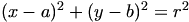((x,y) are the coordinates of any point on the circle, (a,b) is the centre of the circle, r is the radius of the circle). Substitute in the two known points to get: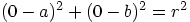,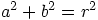,.

Solve these for a to get a=15.

Now you need to draw a diagram (sorry, I realise that this is much more difficult to follow without me drawing one for you, but I need to get back to studying myself). Draw a rough diagram of the circle passing through (0,0) and (30,0) (the centre of the circle is in the first quadrant), being a tangent to y=-5. Also draw the radii to (0,0), (30,0) and to the tangent. Note that for the radius to the tangent, we can say r=b+5 (remember, b is the y coordinate of the centre of the circle, so b is the distance from the centre to the x-axis and 5 is the distance from the x-axis to the circle).
Note also that the coordinates of the point the tangent touches at is (15,-5) (i.e., the x-coordinate is halfway between 0 and 30 - a rough justification is that the -5 is as low as the circle will go, and due to the symmetry this lowest point must lie exactly halfway between any two points with the same y-coordinate, so if y=0 then it lies haflway between x=0 and x=30).

Using these usefel points and Pythagoras' Theorem, we can say that(using a triangle consisting of the points (0,0), (15,0) and the centre of the circle). Remembering that r=b+5,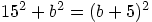, so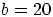. Substitute back intoto get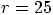, so the equation of the circle is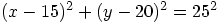.
HMFC - Founded 1874, beefing the Cabbage since 1875

### #3xlynsey

• Gender:Female

Posted 06 May 2007 - 09:01 PM

oh god.. ok. i can just about follow that not that i would ever have thought of that myself!!
i found it in this leckie and leckie questions in higher maths book. this is the first time im used it so im not sure what its like but it seems quite old.
thanks for clearing that up though.
lynsey#### 1 user(s) are reading this topic

0 members, 1 guests, 0 anonymous users